### Home > CCA > Chapter 10 > Lesson 10.2.5 > Problem10-81

10-81.
1. Use square roots to find both solutions to each equation. Homework Help ✎

1. x2 − 81 = 0

2. x2 − 37 = 0

3. x2 + 49 = 0

4. 2x2 + 10 = 0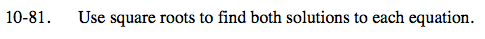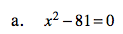Add 81 to both sides.

Square root both sides.

This equation has x2, so you should be looking for two possible solutions.

x = ±9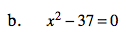Use the same method as part (a). Is 37 a perfect square?

$x=\pm \sqrt{37}$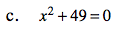Use the same method as parts (a) and (b).

$x=\pm \sqrt{-49}$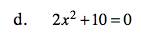One method would be to start by dividing the equation by 2.

$x=\pm \sqrt{-5}$